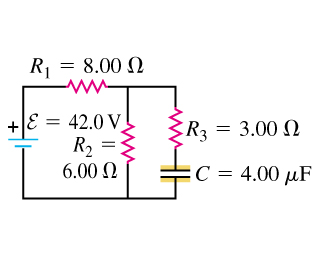# the capacitor in the figure (figure 1)is initially uncharged. the switch is closed at t=0.

edited

The capacitor in the figure (Figure 1) is initially uncharged. The switch is closed at t=0.A- Immediately after the switch is closed, what is the current through the resistor R1?

B- Immediately after the switch is closed, what is the current through the resistor R2?

C- Immediately after the switch is closed, what is the current through each resistor R3?

D- What is the final charge on the capacitor?

selected by

The uncharged cap is like a short at first.

The voltage source "sees" 8+ 6||3 = 10Ω

Therefore there are 42V/10Ω = 4.2A leaving the source.

Above the 6Ω the current divides.

The current in the 6Ω = 4.2*3/(3+6) = 1.4A

The current in the 3Ω = 4.2*6/(3+6) = 2.8A

When the cap is charged, no current flows in the 3Ω

The voltage across the 6Ω = 42*6/(6+8) = 18V which is the voltage across the capacitor after a long time.

Q = CV = 4e-6*18 = 72µC

Lorem ipsum dolor sit amet, consectetur adipiscing elit, sed do eiusmod tempor incididunt ut labore et dolore magna aliqua. Ut enim ad minim veniam, quis nostrud exercitation ullamco laboris nisi ut aliquip ex ea commodo consequat. Duis aute irure dolor in reprehenderit in voluptate velit esse cillum dolore eu fugiat.

Immediately after the switch is closed, the capacitor has no voltage across it, and can be regarded as a short circuit.

R2 in parallel with R3 is 6*3/(6+3) ie 2 ohms.

The voltage across R2 is 42*2/(2+8) = 8.4

The current in R2 is 8.4/3 amps = 2.8 amps (B)

The current in R3 is 8.4/6 amps = 1.4 amps (C)

The current in R1 is the sum of those, ie, 4.2 amps (A)

When the capacitor is fully charged, it draws no current, and no volts are dropped across R3.

The voltage across the capacitor is 42*6/(6+8) = 18 volts

Charge is c*v = 72 uCoulombs (D)

Lorem ipsum dolor sit amet, consectetur adipiscing elit, sed do eiusmod tempor incididunt ut labore et dolore magna aliqua. Ut enim ad minim veniam, quis nostrud exercitation ullamco laboris nisi ut aliquip ex ea commodo consequat. Duis aute irure dolor in reprehenderit in voluptate velit esse cillum dolore eu fugiat.

+1 vote